## Questions

1. The figure below shows how a distant object is focused in a defective eye.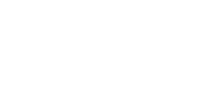1. State the nature of the defect.
2. Suggest suitable lens to correct the defect
2.
1. You are provided with a rectangular glass block, two pins and a piece of white paper. Describe how you would use them to determine the refractive index of the glass using real and apparent image method.
2. An object O is placed 15cm from a converging lens of focal length 10cm.
1. At what distance should a screen be placed so that a focused image is formed on it?
2. A diverging lens of focal length 37.5 cm is placed half way between the converging lens and the screen. How far should the screen be from the diverging leans in order to receive a focused image?
3. Two lenses L1 and L2 placed 12cm from each other. The focal length of L2 is 4cm. An object 5mm high is placed 4cm from L1.
1. Construct a scaled ray diagram on a graph paper to obtain the position of the final image as would be observed by a person on the right hand side of L2
2. Determine the magnification obtained by the arrangement.
3. The figure below represents and object O placed 10cm in front of a diverging lens. F is the focal point of the lens.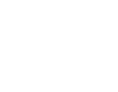Draw rays to locate the position of the image. Determine the image distance.
4. A vertical object is placed at the focal point F of a diverging lens as shown in the figure below.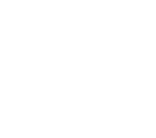Sketch a ray diagram to show the image of the object. (3mks)
5.
1. Describe with the aid of labeled diagram an experiment to determine the focal length of the lens when provided with the following; an illuminated object, a convex lens, a lens holder, a plane mirror and a metre rule. (5mk)
2. A small vertical object is placed 28cm in front of a convex lens of focal length 12cm. On the grid provided, draw a ray diagram to locate the image. The lens position is shown. (Use a scale: 1 cm represents 4 cm).
3. Figure below shows a human eye with a certain defect.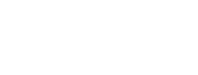1. Name the defect. (1mk)
2. On the same diagram, sketch the appropriate lens to correct the defect and sketch rays to show the effect of the lens. (2mks)
6.
1. Figures (a) and (b) below shows diagrams of the human eye.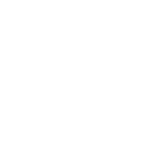1. Sketch in figure (a) a ray diagram to show short sightedness. (1mk)
2. Sketch in figure (b) a ray diagram to show how a lens can be used to correct the shortsightedness. (2mks)
2. The figure below shows the features of a simple camera.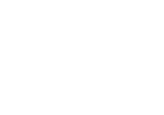1. Name the parts labelled A and B. (2mks)
2. A still object is placed at a certain distance from the camera. Explain the adjustments necessary for a clear image of the object to be formed. (2mks)
3. State the functions of the shutter and the parts labelled A and B (3mks)
3. A lens forms clear image on a screen when the distance between the screen and the object is 80cm. If the image is 3 times the height of the object, determine
1. The distance of the image from the lens. (3mks)
2. The focal length of the lens. (2mks)
7. An image formed on a screen is three times the size of the object. The object and the screen are 80cm apart when the image is sharply focused. Determine the focal length of the lens.
8. A luminous object and a screen are placed on an optical bench a converging lens is placed between them to throw a sharp image of the object on the screen, the magnification is found to be 2.5. The lens is now moved 30cm nearer to the screen and a sharp image is again formed. Calculate the focal length of the lens.
9. An object is placed 16cm from a converging lens of focal length 12cm. Find.
1. Position of image.
2. Nature and
3. Magnification of the image.
10. An object is placed 15cm from a diverging lens and the image is formed 6cm from the lens. What is the focal length of the lens?
11. Calculate the power of a lens whose focal length is given as 10cm.
12. Explain differences between the eye and the camera. State also the similarities.

1.
1. Short sightedness (myopia)
2. Concave lens (diverging lens)
2.
1. Arrange the apparatus as shown below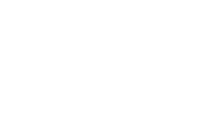Adjust the position of the search pin until there is no parallax. Measure A and R
Refractive index =  Real depth       = R
Apparent depth      A
2.
1. u = 15cm, f = 10cm
1 + 1 = 1
u     v    f
1 = 11 =
v   10  15   30
∴v= 30 cm
2.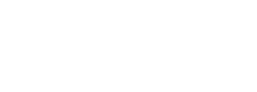- Now for the diverging lens, V= -15cm since the image is vertical
∴ 1 = -1   +   1 (note that for concave is –ve)
v   37.5     15
V= 25 cm
3.
1. Draw rays to form 1st image due to L1. This image will be treated as the object for L2. Use lines (Not rays to obtain the position of the final image I2 then complete the rays as shown below.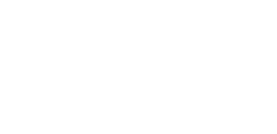2. Measure height of I2 and divide by height of object
3.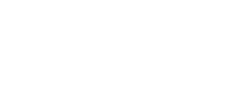4.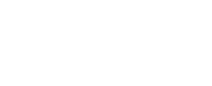5.
1. - Place candle at a distance as shown below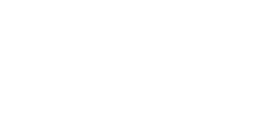- Adjust until a sharp image is formed
- Measure U and V and record
- Repeat this procedure for other values of u
- For each set of values find using 1/f = 1/u + 1/v
- Calculate the average value
2. - Draw one ray from top of object straight through the optical centre of the lens a
- Draw another ray parallel to the principal axis which should pass through principal axis after refraction
- Where they meet, draw the image.
3. Short sightedness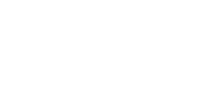6.
1.
1.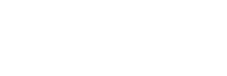2.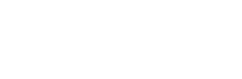2.
1. A-Diaphragm
B-Film
2. The distance between the lens is adjusted so that the image is formed on the film
3. - Shutter- Opens for some given time to alloy rays from the object to fall on the film creating the image impression.
- A (Diaphragm) controls intensity of light entering the camera
- B (Film) – coated with light sensitive components which react with light to create the impression
3.
1. Magnification = v = 3
u
Since v + u = 80
u = 80 – v
v      = 3
80 – v
v= 240 – 3v
v= 60 cm
2. From above u = 20cm
1= 1 + 1 + 1 + 1
F    v    u    60  20
7. f= 15cm
8. f= 14.3cm
9.
1. Given information
u= 16cm
f = 12cm
Applying 1 = 1 + 1
f     u    v
then 1= 1 + 1
v   f     u
1=  11
v    12  16
= 1 = 4 -3 = 1
v     48     48
Hence image distance = 48
2. Nature: image is real
- Inverted
- 48 cm from the lens
- Magnified
3. Magnification, m= v = 48 cm = 3
u    16 cm
10. Solution
Given information
v= -6 cm
u = + 15cm
Applying 1 = 1 + 1
f     u    v
1=  1 – 1
f   15    6
1 2 – 5
f       30
1– 3
f     30
1– 1
f     10
f = -10
f= 10 cm
11. Solution
P = 1/f
P =   1        =      1
10 cm          10/100m
= 1÷ 10/100 m
= 1 x 100/10
Power = 10 dioptre (D)
12. Similarity
- Both have converging lens
- In the eye, retina act as the screen while in the camera a light sensitive film act as one

Differences
- Focal length of the eye lens is variable while that of the camera is fixed.

• ✔ To read offline at any time.
• ✔ To Print at your convenience
• ✔ Share Easily with Friends / Students